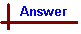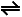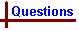Equilibrium - Calculations with Complexes Link to Data Page Your feedback on these self-help problems is appreciated. Click here to send an e-mail. Shortcut to Questions Q: 1 2 3 4 5 1 Give the formula for and arrange the following complex ions in order of increasing stability: tetramminecopper(II) ion tetracyanomercurate(II) ion tetramminezinc ion tetracyanocadmate ion2 A solution is prepared by mixing 0.0200 M silver nitrate (10.0 cm3) with 1.04 M sodium cyanide (10.0 cm3). (1.) Calculate the resulting concentration of silver ion. (2.) Predict what effect, if any, will occur if 1.00 M ammonia (1.00 cm3) is added to the solution.3 What is the concentration of copper(II) ion in a solution made by dissolving copper(II) sulfate (0.100 mole) and ammonia (2 .00 mole) in water and making up to 500 mL?4 (Advanced question) Unexposed silver halides are removed from photographic film when they react with a solution of Na2S2O3 and form the complex Ag(S2O3)23-. What mass of sodium thiosulfate is required to prepare 1.00 L of a solution that will dissolve 1.00 g of silver bromide by the formation of this complex?5 (Advanced question) Slow addition of sodium iodide to a mercury(II) nitrate solution results initially in the precipitation of red mercury(II) iodide and then the dissolution of this salt as a result of the reaction HgI2(s) + 2I-[HgI4]2- (1.) Using Kstab for [HgI4]2- (= 1030.28) and Kso for HgI2 (=10-10.37), calculate the equilibrium constant for this reaction. (2.) To 1.00 L of sodium iodide solution (0.200 M) is added 10.0 g of solid Hg(NO3)2. ½H2O. Calculate the final concentration of free (uncomplexed) mercury(II) ion? (3.) Will solid mercury(II) iodide be present at equilibrium in the above reaction?Equilibrium - Calculations with Complexes (Answers) 1 Stability is quantified by the compound's KSTAB (the stability constant) which is an expression incorporating the ratio of the concentration of the compex and the concentrations of the dissociated metal and ligand species. It always has the form KSTAB = [complex] / ([metal ion][ligand]n) where n is the number of ligand species in the complex. The larger its KSTAB value, the more associated the complex - i.e. the more stable it is. Since the complexes in the question all have the same number of ligands (all have n = 4), their KSTAB values have the same units, and can be compared directly. Using KSTAB values from the data sheet the complexes are listed in the following order of increasing stability: Zn(NH3)42+ (KSTAB = 1 x 109 M-4) Cu(NH3)42+ (KSTAB = 1 x 1013 M-4) Cd(CN)42- (KSTAB = 1 x 1018 M-4) Hg(CN)42- (KSTAB = 1 x 1041 M-4)2 (1.) The silver and cyanide ions will react to form the [Ag(CN)2]- complex: Ag+ + 2CN-[Ag(CN)2]- KSTAB = 1 x 1020 M-2 Note the use of square brackets in a reaction equation to indicate a complex ion - square brackets are also used in concentration calculations to denote concentration of a species (this can be confusing!) The amount of free Ag+ left in solution is found by using the KSTAB of this complex. The final volume is 20 mL, which is twice the volume of each of the added solutions, so their concentration in the mixed solution needs to be corrected by a factor of 0.50: ∴ [Ag+]init = 0.500 x 0.0200 M = 0.0100 M ∴ [CN-]init = 0.500 x 1.04 M = 0.520 M Next the equlibrium concentrations are calculated: From the reaction equation we see that each mole of Ag+ reacts with two moles of CN-, so the equilibrium concentration of CN- is found: [CN-]eqb = 0.520 mol L-1 - (2 x 0.0100 M) = 0.500 M We assume all Ag+ initially present reacts to form the complex [Ag(CN)2-], so [Ag(CN)2-]eqb = [Ag+]init = 0.0100 M Now KSTAB = [Ag(CN)2-] / ([Ag+] [CN-]2) = 1 x 1020 at equilibrium. Rearranging the above expression for KSTAB: [Ag+] = [[Ag(CN)2-]] / KSTAB [CN-]2 = 0.0100 / (1 x 1020 ) x (0.50 )2 = 4 x 10-22 M (2.) The addition of NH3 solution will produce no effect since the KSTAB of [Ag(CN)2]- (1 x 1020 M-2) is much larger than that of [Ag(NH3)2]+ (1 x 107 M-2)3 First the initial concentrations of Cu2+ and NH3 are obtained: [Cu2+]init = 0.200 M [NH3]init = 4.00 M They react according to the equation: Cu2+ + 4NH3[Cu(NH3)4]2+ KSTAB = 1 x 1013 M-4 From this equation we see that each mole of Cu2+ reacts with 4 moles of NH3, so the equlibrium concentration of NH3 is calculated: [NH3]eqb = 4.00 - (4 x 0.200) = 3.20 M We assume all Cu2+ initially present reacts to form the complex [Cu(NH3)4]2+, so [Cu(NH3)4]2+eqb = [Cu2+]init = 0.200 M. (This is typically justified by the stability constant of complexes being very large). Now KSTAB = [Cu(NH3)42+] / [Cu2+] [NH3]4 = 1 x 1013 M at equilibrium. Rearranging for [Cu2+]: [Cu2+] = [Cu(NH3)42+] / KSTAB [NH3]4 = 0.2 00/ (1 x 1013 x (3.20)4) = 2 x 10-16 M4 KSP(AgBr) = [Ag+] [Br-] = 5.0 x 10-13 KSTAB(Ag(S2O3)23-) = [Ag(S2O3)23-] / ([Ag+] [S2O32-]2) = 1013.5 KSP x KSTAB = 5.0 x 10-13 x 1013.5 = 5.0 x 100.5 = 15.81 = ([Br-] [Ag(S2O3)23-]) / [S2O32-]2 Let x = [Ag+] at equilibrium Let y = initial [S2O32-] At equilibrium: [Br-] = 5.32 x 10-3 M [Ag(S2O3)23-] = (5.32 x 10-3 - x) M [S2O32-] = y - 2(5.32 x 10-3 - x) M ∴ 15.81 = (5.32 x 10-3) (5.32 x 10-3 - x) / ((y - 2(5.32 x 10-3 - x)2) Now we can assume that x << 5.32 x 10-3, since practically all silver is complexed at equilibrium (ie KSTAB(Ag(S2O3)23-) is very large): ∴ y - 10.64 x 10-3 = ((5.32 x 10-3) (5.32 x 10-3) / 15.81)½ = 5.32 x 10-3 / 3.976 = 1.34 x 10-3 y = 1.34 x 10-3 + 10.64 x 10-3 = 1.198 x 10-2 M ∴ Mass of Na2S2O3 required for 1.00 L of solution = 1.00 x 1.198 x 10-2 x 158.1 = 2 g (to 1 significant figure as required by the precision of the KSTAB(Ag(S2O3)23-)).5 (1.) HgI2(s) + 2I-HgI42- Hg2+ + 2I-HgI2(s) K for HgI2(s) + 2I-HgI42- needs to be found, ie. [HgI42-] / [I-]2 KSTAB(HgI42-) = [HgI42-] / ([Hg2+] [I-]4) = 1030.28 KSP(HgI2) = [Hg2+] [I-]2 KSTAB x KSP = [HgI42-] / [I-]2 = 1030.28 x 10-10.37 = 1019.91 ∴ K = 1019.91 (2.) The reaction is Hg2+ + 4I-HgI42- Initial [Hg2+] = moles Hg(NO3)2. ½H2O / volume (L) Volume = 1.00 L, so Initial [Hg2+] = mass / molar mass Hg(NO3)2. ½H2O = 10.0 / 333.6 M Inital [I-] = 0.200 M Inital [HgI42-] = 0 Equilibrium [Hg2+] = x Equilibrium [I-] = 0.20 - 4(10/333.6 - x) = 8.0 x 10-2 M (assuming x << 10/333.6, and ignoring it) Equilibrium [HgI42-] = 10/333.6 - x = 3.0 x 10-2 M (assuming x << 10/333.6, and ignoring it) KSTAB = (3.0 x 10-2) / (x(8 x 10-2)4) = 1030.28 x = (3.0 x 10-2) / (1030.28 x 4.1 x 10-5) = 7.32 x 102 / 1030.28 = 3.8 x 10-28 M (3.) Q = [Hg2+] [I-]2 = (3.8 x 10-28) (8.0 x 10-2)2 = 2.5 x 10-30. This is less than KSP(HgI2), so no precipitate will form.